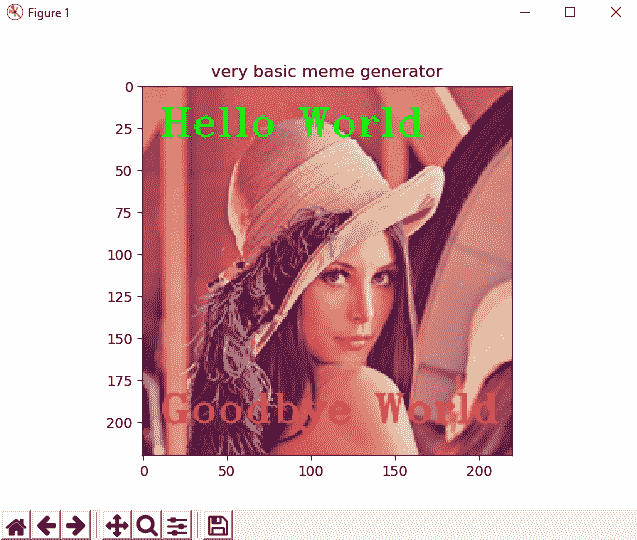# 四、在 OpenCV 中构造基本形状

OpenCV 提供的一种基本功能是绘制基本形状。 OpenCV 提供绘制线，圆，矩形，椭圆等的函数。 在构建计算机视觉项目时，通常需要通过绘制一些形状来修改图像。 例如，如果开发人脸检测算法，则应绘制一个矩形以突出显示在计算图像中检测到的人脸。 此外，如果您开发了面部识别算法，则应绘制一个矩形突出显示检测到的面部，并编写一些文本来显示检测到的面部的身份。 最后，写一些调试信息是一种常见的方法。 例如，您可以显示检测到的脸部数量（以便查看脸部检测算法的表现）或处理时间。 在本章中，您将了解如何使用 OpenCV 库绘制基本的和更高级的形状。

• OpenCV 中绘图的理论介绍
• 基本形状 - 直线，矩形和圆形
• 基本形状（2）- 直线和箭头线，椭圆和折线
• 绘制文字
• 带有鼠标事件的动态绘图
• 高级绘图

# 技术要求

• Python 和 OpenCV
• 特定于 Python 的 IDE
• NumPy 和 Matplotlib 包
• Git 客户端

# OpenCV 中绘图的理论介绍

OpenCV 提供了许多绘制基本形状的函数。 常见的基本形状包括直线，矩形和圆形。 但是，使用 OpenCV，我们可以绘制更多基本形状。 如引言中简要提到的，这是在图像上绘制基本形状以执行以下操作的常用方法：

• 显示算法的一些中间结果
• 显示算法的最终结果
• 显示一些调试信息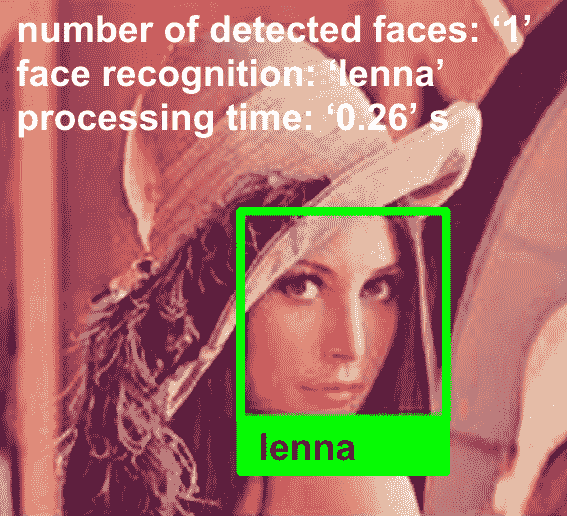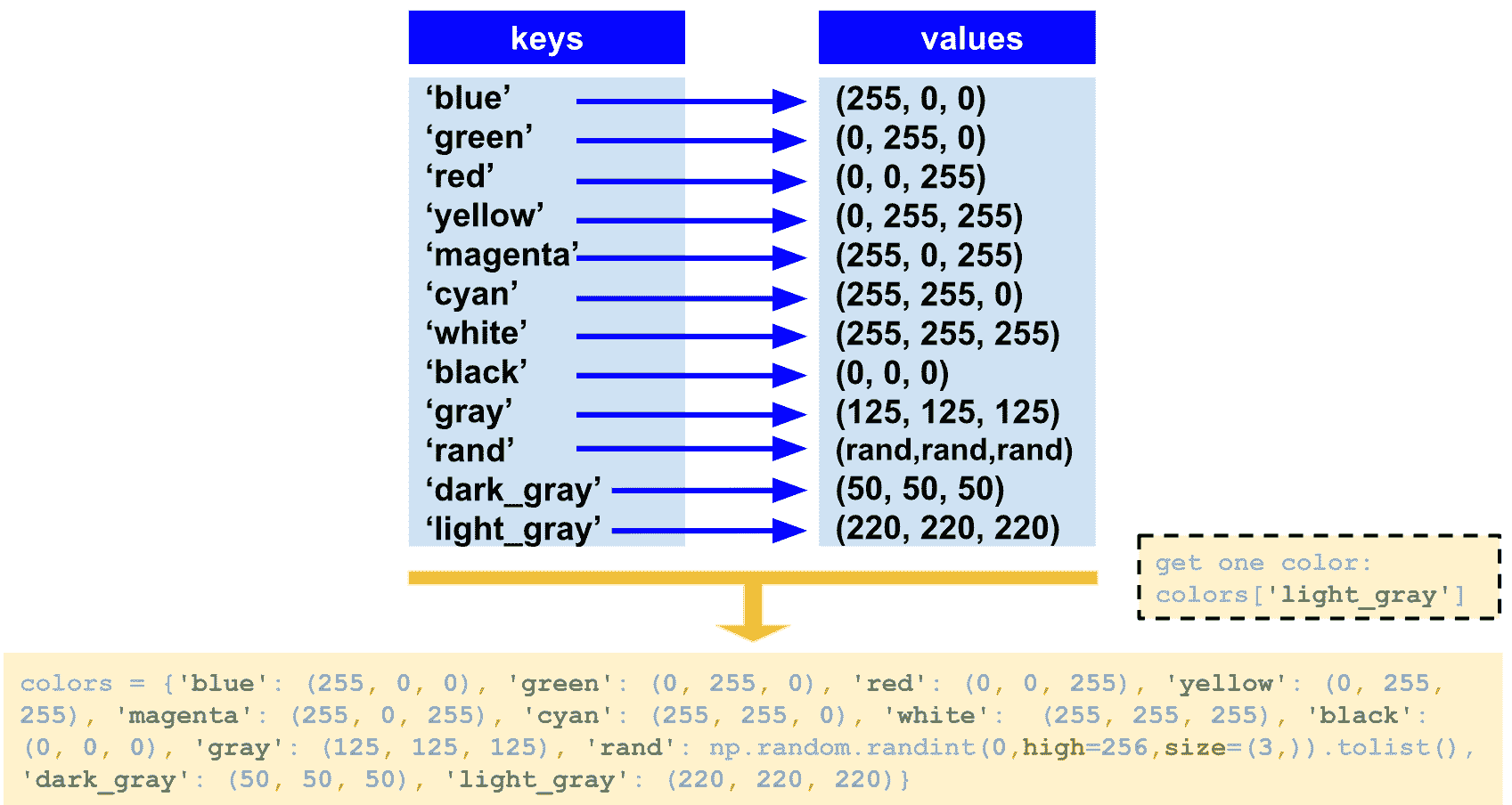"""
Common colors triplets (BGR space) to use in OpenCV
"""

BLUE = (255, 0, 0)
GREEN = (0, 255, 0)
RED = (0, 0, 255)
YELLOW = (0, 255, 255)
MAGENTA = (255, 0, 255)
CYAN = (255, 255, 0)
DARK_GRAY = (50, 50, 50)
...


import constant

# Getting red color:
print("red: '{}'".format(constant.RED))


# Dictionary containing some colors
colors = {'blue': (255, 0, 0), 'green': (0, 255, 0), 'red': (0, 0, 255), 'yellow': (0, 255, 255), 'magenta': (255, 0, 255), 'cyan': (255, 255, 0), 'white': (255, 255, 255), 'black': (0, 0, 0), 'gray': (125, 125, 125), 'rand': np.random.randint(0, high=256, size=(3,)).tolist(), 'dark_gray': (50, 50, 50), 'light_gray': (220, 220, 220)}


colors['magenta']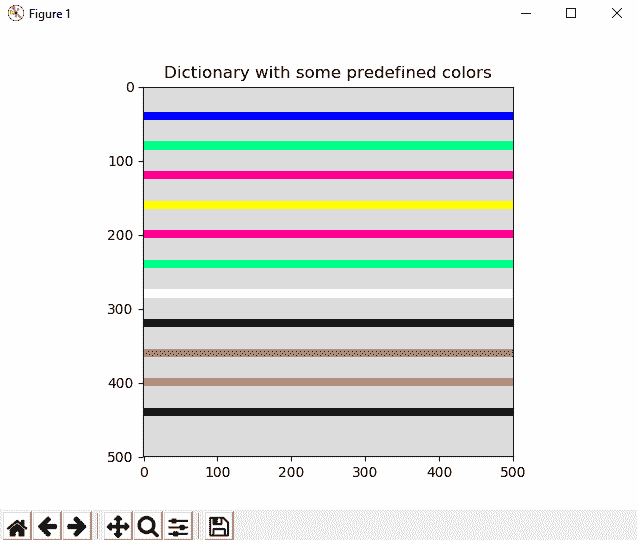# We create the canvas to draw: 400 x 400 pixels, 3 channels, uint8 (8-bit unsigned integers)
# We set background to black using np.zeros()
image = np.zeros((500, 500, 3), dtype="uint8")


# If you want another background color, you can do the following:
image[:] = colors['light_gray']


# We draw all the colors to test the dictionary
# We draw some lines, each one in a color. To get the color, use 'colors[key]'
separation = 40
for key in colors:
cv2.line(image, (0, separation), (500, separation), colors[key], 10)
separation += 40


# Show image:
show_with_matplotlib(image, 'Dictionary with some predefined colors')


show_with_matplotlib()的两个参数是要绘制的图像和要显示的标题。 因此，现在我们准备开始使用 OpenCV 和 Python 创建一些基本形状。

# 基本形状 – 直线，矩形和圆形

# We create the canvas to draw: 400 x 400 pixels, 3 channels, uint8 (8-bit unsigned integers)
# We set the background to black using np.zeros()
image = np.zeros((400, 400, 3), dtype="uint8")


# If you want another background color, you can do the following:
image[:] = colors['light_gray']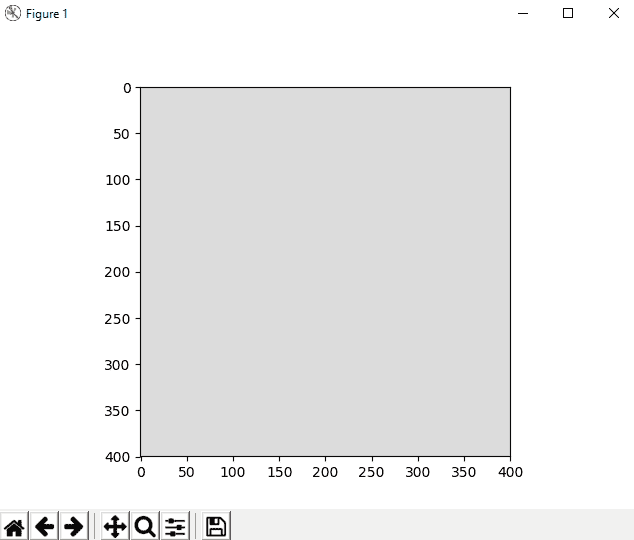• img：是要绘制形状的图像。
• color：它是用来绘制形状的颜色（BGR 三元组）。
• thickness：如果该值为正，则是形状轮廓的厚度。 否则，将绘制填充形状。
• lineType：这是形状边界的类型。 OpenCV 提供三种类型的线：
• cv2.LINE_4：这表示四线连接
• cv2.LINE_8：这表示八连接线
• cv2.LINE_AA：这表示抗锯齿线
• shift：这表示与定义形状的某些点的坐标有关的小数位数。

# 绘制直线

img = line(img, pt1, pt2, color, thickness=1, lineType=8, shift=0)


cv2.line(image, (0, 0), (400, 400), colors['green'], 3)
cv2.line(image, (0, 400), (400, 0), colors['blue'], 10)
cv2.line(image, (200, 0), (200, 400), colors['red'], 3)
cv2.line(image, (0, 200), (400, 200), colors['yellow'], 10)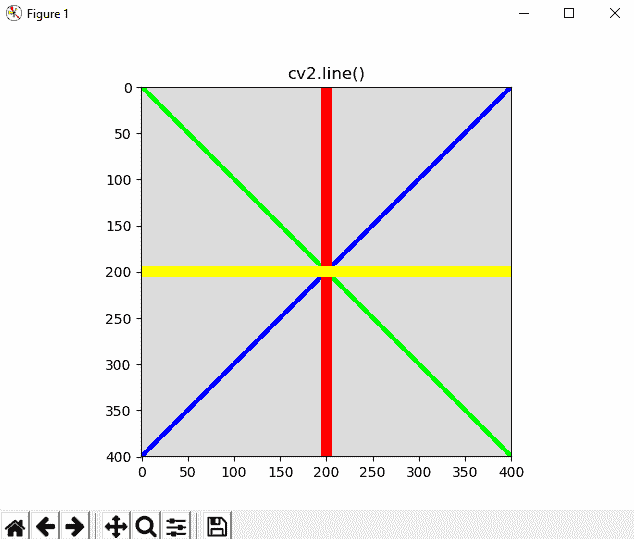# 绘制矩形

cv2.rectangle()函数的签名如下：

img = rectangle(img, pt1, pt2, color, thickness=1, lineType=8, shift=0)


cv2.rectangle(image, (10, 50), (60, 300), colors['green'], 3)
cv2.rectangle(image, (80, 50), (130, 300), colors['blue'], -1)
cv2.rectangle(image, (150, 50), (350, 100), colors['red'], -1)
cv2.rectangle(image, (150, 150), (350, 300), colors['cyan'], 10)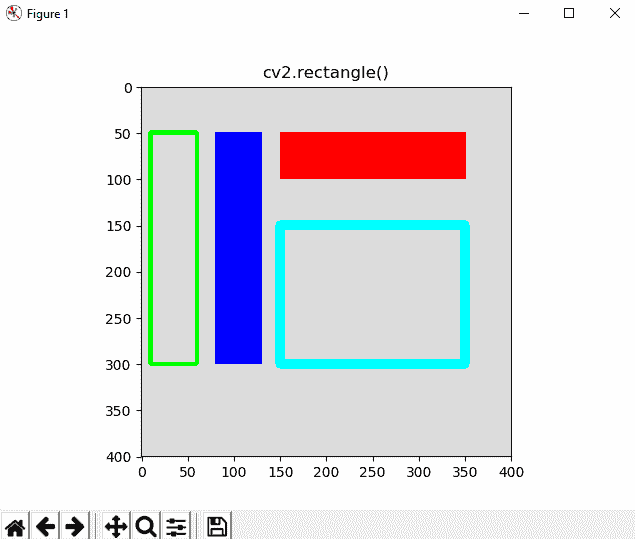# 绘制圆形

cv2.circle()函数的签名如下：

img = circle(img, center, radius, color, thickness=1, lineType=8, shift=0)


cv2.circle(image, (50, 50), 20, colors['green'], 3)
cv2.circle(image, (100, 100), 30, colors['blue'], -1)
cv2.circle(image, (200, 200), 40, colors['magenta'], 10)
cv2.circle(image, (300, 300), 40, colors['cyan'], -1)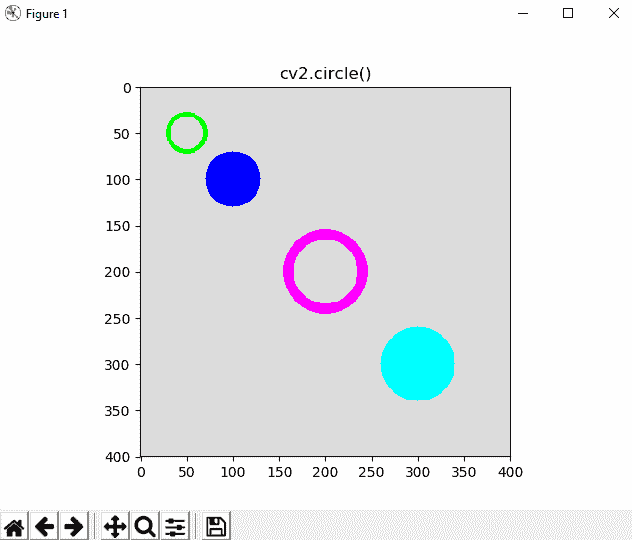# 了解高级形状

# We create the canvas to draw: 300 x 300 pixels, 3 channels, uint8 (8-bit unsigned integers)
# We set the background to black using np.zeros()
image = np.zeros((300, 300, 3), dtype="uint8")


# If you want another background color, you can do the following:
image[:] = colors['light_gray']


# 绘制剪切线

cv2.clipLine()函数的签名如下：

retval, pt1, pt2 = clipLine(imgRect, pt1, pt2)


cv2.clipLine()函数返回矩形内的段（由pt1pt2输出点定义）（函数根据定义的矩形剪切片段）。 从这个意义上讲，如果两个原始pt1pt2点都在矩形外部，则retvalFalse。 否则（两个pt1pt2点中的一些在矩形内）此函数返回True。 在下一段代码中可以更清楚地看到这一点：

cv2.line(image, (0, 0), (300, 300), colors['green'], 3)
cv2.rectangle(image, (0, 0), (100, 100), colors['blue'], 3)
ret, p1, p2 = cv2.clipLine((0, 0, 100, 100), (0, 0), (300, 300))
if ret:
cv2.line(image, p1, p2, colors['yellow'], 3)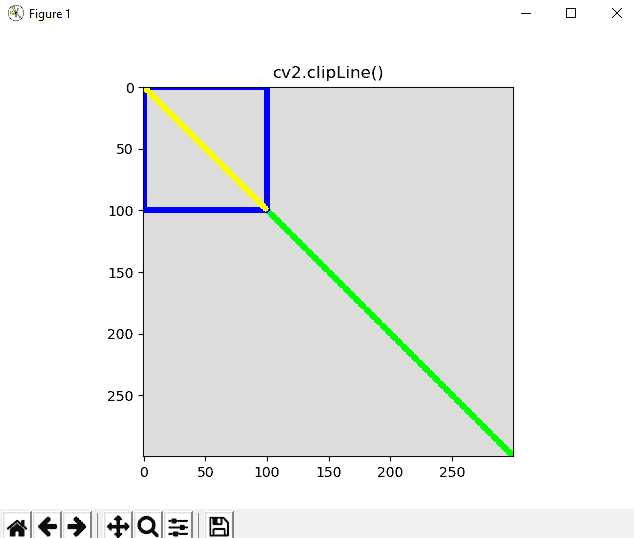# 绘制箭头

cv.arrowedLine(img, pt1, pt2, color, thickness=1, lineType=8, shift=0, tipLength=0.1)


cv2.arrowedLine(image, (50, 50), (200, 50), colors['red'], 3, 8, 0, 0.1)
cv2.arrowedLine(image, (50, 120), (200, 120), colors['green'], 3, cv2.LINE_AA, 0, 0.3)
cv2.arrowedLine(image, (50, 200), (200, 200), colors['blue'], 3, 8, 0, 0.3)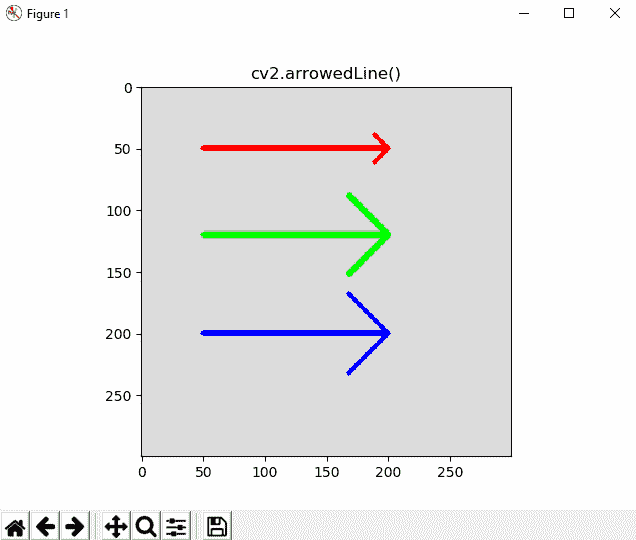# 绘制椭圆

cv2.ellipse(img, center, axes, angle, startAngle, endAngle, color, thickness=1, lineType=8, shift=0)


cv2.ellipse(image, (80, 80), (60, 40), 0, 0, 360, colors['red'], -1)
cv2.ellipse(image, (80, 200), (80, 40), 0, 0, 360, colors['green'], 3)
cv2.ellipse(image, (80, 200), (10, 40), 0, 0, 360, colors['blue'], 3)
cv2.ellipse(image, (200, 200), (10, 40), 0, 0, 180, colors['yellow'], 3)
cv2.ellipse(image, (200, 100), (10, 40), 0, 0, 270, colors['cyan'], 3)
cv2.ellipse(image, (250, 250), (30, 30), 0, 0, 360, colors['magenta'], 3)
cv2.ellipse(image, (250, 100), (20, 40), 45, 0, 360, colors['gray'], 3)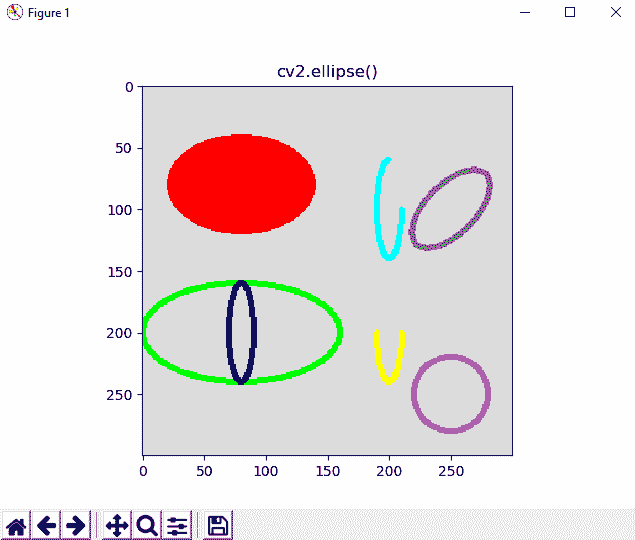# 绘制多边形

cv2.polylines(img, pts, isClosed, color, thickness=1, lineType=8, shift=0)


# These points define a triangle
pts = np.array([[250, 5], [220, 80], [280, 80]], np.int32)
# Reshape to shape (number_vertex, 1, 2)
pts = pts.reshape((-1, 1, 2))
# Print the shapes: this line is not necessary, only for visualization
print("shape of pts '{}'".format(pts.shape))
# this gives: shape of pts '(3, 1, 2)'


# These points define a triangle
pts = np.array([[250, 5], [220, 80], [280, 80]], np.int32)
# Reshape to shape (number_vertex, 1, 2)
pts = pts.reshape((-1, 1, 2))
# Print the shapes: this line is not necessary, only for visualization
print("shape of pts '{}'".format(pts.shape))
# Draw this poligon with True option
cv2.polylines(image, [pts], True, colors['green'], 3)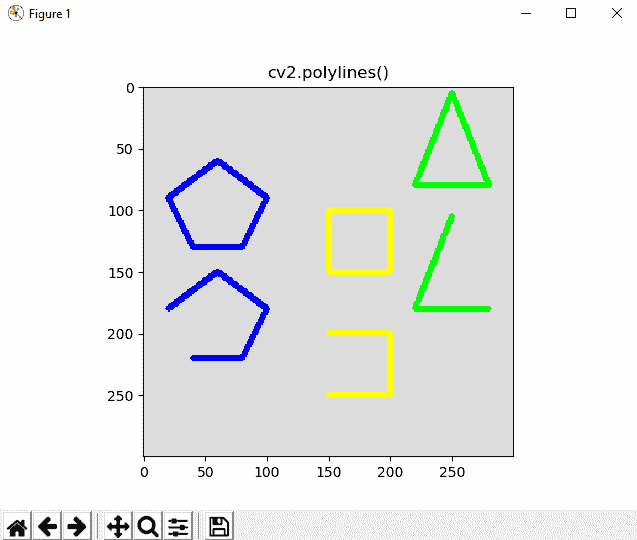# 绘图函数中的shift参数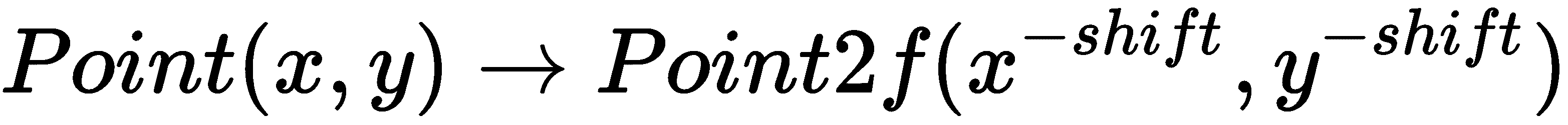shift = 2
factor = 2 ** shift
print("factor: '{}'".format(factor))
cv2.circle(image, (int(round(299.99 * factor)), int(round(299.99 * factor))), 300 * factor, colors['red'], 1, shift=shift)
cv2.circle(image, (299, 299), 300, colors['green'], 1)


def draw_float_circle(img, center, radius, color, thickness=1, lineType=8, shift=4):
"""Wrapper function to draw float-coordinate circles

"""
factor = 2 ** shift
center = (int(round(center * factor)), int(round(center * factor)))
cv2.circle(img, center, radius, color, thickness, lineType, shift)

draw_float_circle(image, (299, 299), 300, colors['red'], 1, 8, 0)
draw_float_circle(image, (299.9, 299.9), 300, colors['green'], 1, 8, 1)
draw_float_circle(image, (299.99, 299.99), 300, colors['blue'], 1, 8, 2)
draw_float_circle(image, (299.999, 299.999), 300, colors['yellow'], 1, 8, 3)


# 绘图函数中的lineType参数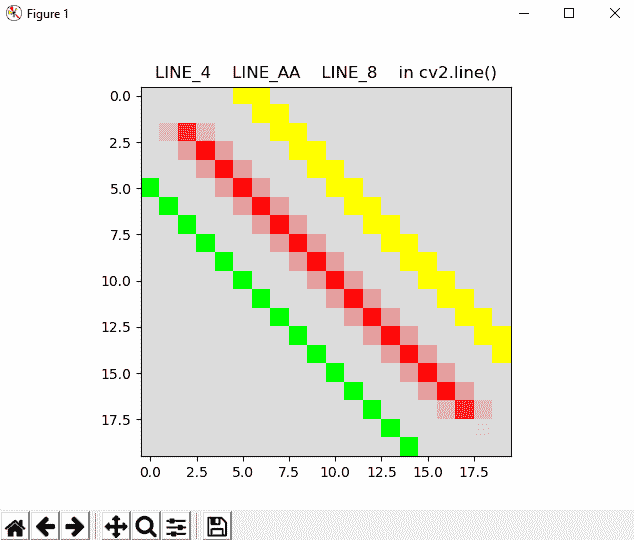# 绘制文字

OpenCV 还可以用于在图像中呈现文本。 在本节中，我们将看到如何使用cv2.putText()函数绘制文本。 此外，我们将看到您可以使用的所有可用字体。 最后，我们将看到一些与文本绘制有关的 OpenCV 函数。

# 绘制文字

cv2.putText()函数具有以下签名：

img = cv.putText( img, text, org, fontFace, fontScale, color, thickness=1, lineType= 8, bottomLeftOrigin=False)


# We draw some text on the image:
cv2.putText(image, 'Mastering OpenCV4 with Python', (10, 30), cv2.FONT_HERSHEY_SIMPLEX, 0.9, colors['red'], 2, cv2.LINE_4)
cv2.putText(image, 'Mastering OpenCV4 with Python', (10, 70), cv2.FONT_HERSHEY_SIMPLEX, 0.9, colors['red'], 2, cv2.LINE_8)
cv2.putText(image, 'Mastering OpenCV4 with Python', (10, 110), cv2.FONT_HERSHEY_SIMPLEX, 0.9, colors['red'], 2, cv2.LINE_AA)

# Show image:
show_with_matplotlib(image, 'cv2.putText()')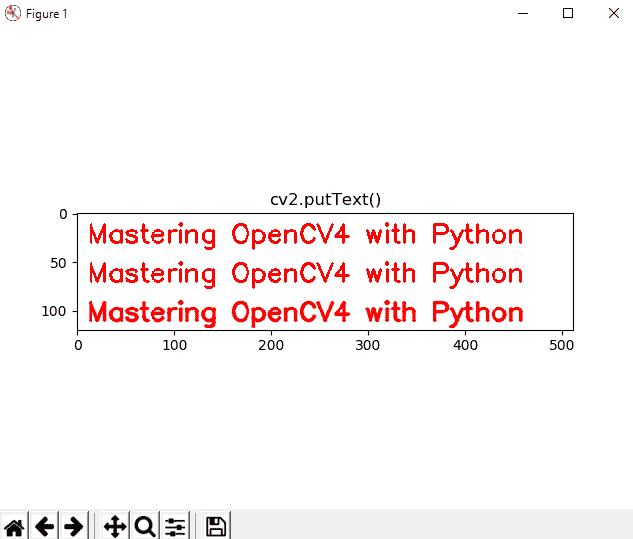image.fill(255)


# 使用所有 OpenCV 文本字体

OpenCV 中所有可用的字体如下：

• FONT_HERSHEY_SIMPLEX = 0
• FONT_HERSHEY_PLAIN = 1
• FONT_HERSHEY_DUPLEX = 2
• FONT_HERSHEY_COMPLEX = 3
• FONT_HERSHEY_TRIPLEX = 4
• FONT_HERSHEY_COMPLEX_SMALL = 5
• FONT_HERSHEY_SCRIPT_SIMPLEX = 6
• FONT_HERSHEY_SCRIPT_COMPLEX = 7

position = (10, 30)
for i in range(0, 8):
cv2.putText(image, fonts[i], position, i, 1.1, colors[index_colors[i]], 2, cv2.LINE_4)
position = (position, position + 40)
cv2.putText(image, fonts[i].lower(), position, i, 1.1, colors[index_colors[i]], 2, cv2.LINE_4)
position = (position, position + 40)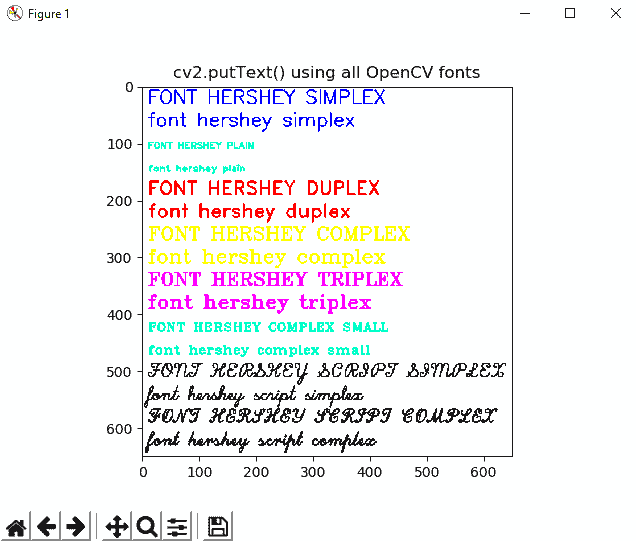# 与文字相关的更多函数

OpenCV 提供了更多与文本绘制有关的函数。 应当注意，这些函数不是用于绘制文本，而是可以用于对上述cv2.putText()函数进行补充，它们的评论如下。 我们要看到的第一个函数是cv2.getFontScaleFromHeight()。 该函数的签名如下：

retval = cv2.getFontScaleFromHeight(fontFace, pixelHeight, thickness=1)


retval, baseLine = cv2.getTextSize(text, fontFace, fontScale, thickness)


# assign parameters to use in the drawing functions
font = cv2.FONT_HERSHEY_SIMPLEX
font_scale = 2.5
thickness = 5
text = 'abcdefghijklmnopqrstuvwxyz'

# We get the size of the text
ret, baseline = cv2.getTextSize(text, font, font_scale, thickness)

# We get the text width and text height from ret
text_width, text_height = ret

# We center the text in the image
text_x = int(round((image.shape - text_width) / 2))
text_y = int(round((image.shape + text_height) / 2))

# Draw this point for reference:
cv2.circle(image, (text_x, text_y), circle_radius, colors['green'], -1)

# Draw the rectangle (bounding box of the text)
cv2.rectangle(image, (text_x, text_y + baseline), (text_x + text_width - thickness, text_y - text_height),
colors['blue'], thickness)

# Draw the circles defining the rectangle
cv2.circle(image, (text_x, text_y + baseline), circle_radius, colors['red'], -1)
cv2.circle(image, (text_x + text_width - thickness, text_y - text_height), circle_radius, colors['cyan'], -1)

# Draw the baseline line
cv2.line(image, (text_x, text_y + int(round(thickness/2))), (text_x + text_width - thickness, text_y +
int(round(thickness/2))), colors['yellow'], thickness)
# Write the text centered in the image
cv2.putText(image, text, (text_x, text_y), font, font_scale, colors['magenta'], thickness)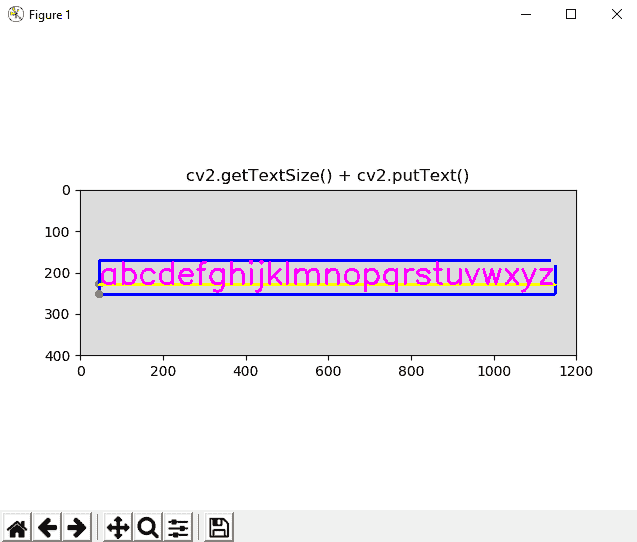# 绘制动态形状

cv2.setMouseCallback(windowName, onMouse, param=None)


# This is the mouse callback function:
def draw_circle(event, x, y, flags, param):
if event == cv2.EVENT_LBUTTONDBLCLK:
print("event: EVENT_LBUTTONDBLCLK")
cv2.circle(image, (x, y), 10, colors['magenta'], -1)

if event == cv2.EVENT_MOUSEMOVE:
print("event: EVENT_MOUSEMOVE")

if event == cv2.EVENT_LBUTTONUP:
print("event: EVENT_LBUTTONUP")

if event == cv2.EVENT_LBUTTONDOWN:
print("event: EVENT_LBUTTONDOWN")


draw_circle()函数接收特定事件以及每个鼠标事件的坐标(x, y)。 在这种情况下，当执行左键双击（cv2.EVENT_LBUTTONDBLCLK）时，我们在事件的相应(x, y)坐标中绘制一个圆。

# We create a named window where the mouse callback will be established
cv2.namedWindow('Image mouse')


# We set the mouse callback function to 'draw_circle'
cv2.setMouseCallback('Image mouse', draw_circle)


# 绘制文字和形状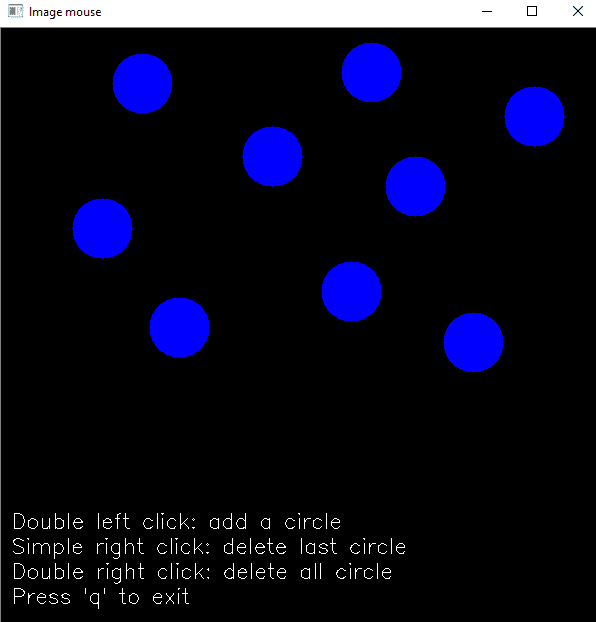• 使用鼠标左键双击添加一个圆
• 只需单击鼠标左键即可删除最后添加的圆圈
• 双击右键删除所有圆圈

# Matplotlib 事件处理

# 'button_press_event' is a MouseEvent where a mouse botton is click (pressed)
# When this event happens the function 'click_mouse_event' is called:
figure.canvas.mpl_connect('button_press_event', click_mouse_event)


# We define the event listener for the 'button_press_event':
def click_mouse_event(event):
# (event.xdata, event.ydata) contains the float coordinates of the mouse click event:
cv2.circle(image, (int(round(event.xdata)), int(round(event.ydata))), 30, colors['blue'], cv2.FILLED)
# Call 'update_image()' method to update the Figure:
update_img_with_matplotlib()


# 高级绘图

• analog_clock_values.py
• analog_clock_opencv.py

analog_clock_opencv.py脚本使用cv.line()cv.circle()cv.rectangle()cv2.putText()绘制模拟时钟。 在此脚本中，我们首先绘制静态图形。 从这个意义上讲，您可以看到有两个数组包含固定的坐标：

hours_orig = np.array(
[(620, 320), (580, 470), (470, 580), (320, 620), (170, 580), (60, 470), (20, 320), (60, 170), (169, 61), (319, 20),
(469, 60), (579, 169)])

hours_dest = np.array(
[(600, 320), (563, 460), (460, 562), (320, 600), (180, 563), (78, 460), (40, 320), (77, 180), (179, 78), (319, 40),
(459, 77), (562, 179)])


for i in range(0, 12):
cv2.line(image, array_to_tuple(hours_orig[i]), array_to_tuple(hours_dest[i]), colors['black'], 3)


cv2.circle(image, (320, 320), 310, colors['dark_gray'], 8)


cv2.rectangle(image, (150, 175), (490, 270), colors['dark_gray'], -1)
cv2.putText(image, "Mastering OpenCV 4", (150, 200), 1, 2, colors['light_gray'], 1, cv2.LINE_AA)
cv2.putText(image, "with Python", (210, 250), 1, 2, colors['light_gray'], 1, cv2.LINE_AA)


image_original = image.copy()


1. 获取当前时间的小时，分​​钟和秒：
# Get current date:
date_time_now = datetime.datetime.now()
# Get current time from the date:
time_now = date_time_now.time()
# Get current hour-minute-second from the time:
hour = math.fmod(time_now.hour, 12)
minute = time_now.minute
second = time_now.second

1. 将这些值（小时，分钟和秒）转换为角度：
# Get the hour, minute and second angles:
second_angle = math.fmod(second * 6 + 270, 360)
minute_angle = math.fmod(minute * 6 + 270, 360)
hour_angle = math.fmod((hour*30) + (minute/2) + 270, 360)

1. 绘制与时针，分针和秒针相对应的线：
# Draw the lines corresponding to the hour, minute and second needles:
second_x = round(320 + 310 * math.cos(second_angle * 3.14 / 180))
second_y = round(320 + 310 * math.sin(second_angle * 3.14 / 180))
cv2.line(image, (320, 320), (second_x, second_y), colors['blue'], 2)

minute_x = round(320 + 260 * math.cos(minute_angle * 3.14 / 180))
minute_y = round(320 + 260 * math.sin(minute_angle * 3.14 / 180))
cv2.line(image, (320, 320), (minute_x, minute_y), colors['blue'], 8)

hour_x = round(320 + 220 * math.cos(hour_angle * 3.14 / 180))
hour_y = round(320 + 220 * math.sin(hour_angle * 3.14 / 180))
cv2.line(image, (320, 320), (hour_x, hour_y), colors['blue'], 10)

1. 最后，绘制一个小圆圈，对应于三个针的连接点：
cv2.circle(image, (320, 320), 10, colors['dark_gray'], -1)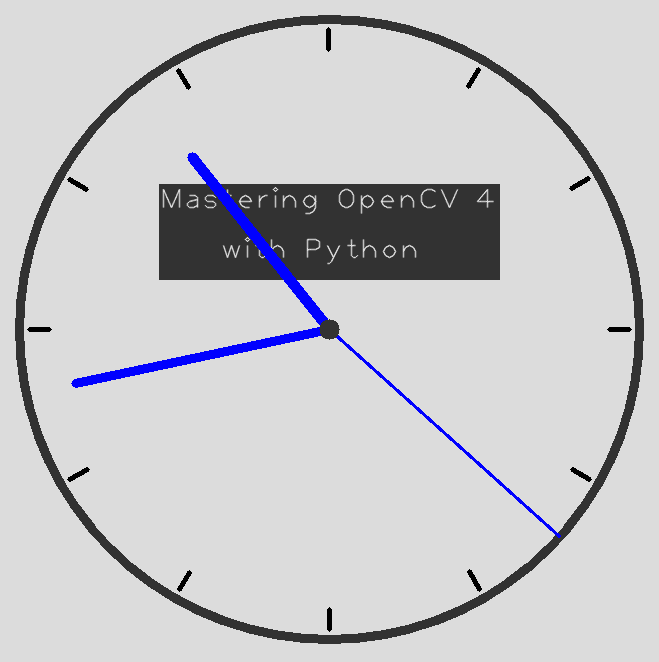script analog_clock_values.py脚本计算hours_orighours_dest数组的固定坐标。 要计算小时标记的(x, y)坐标，我们使用圆的参数方程式，如下面的屏幕截图所示：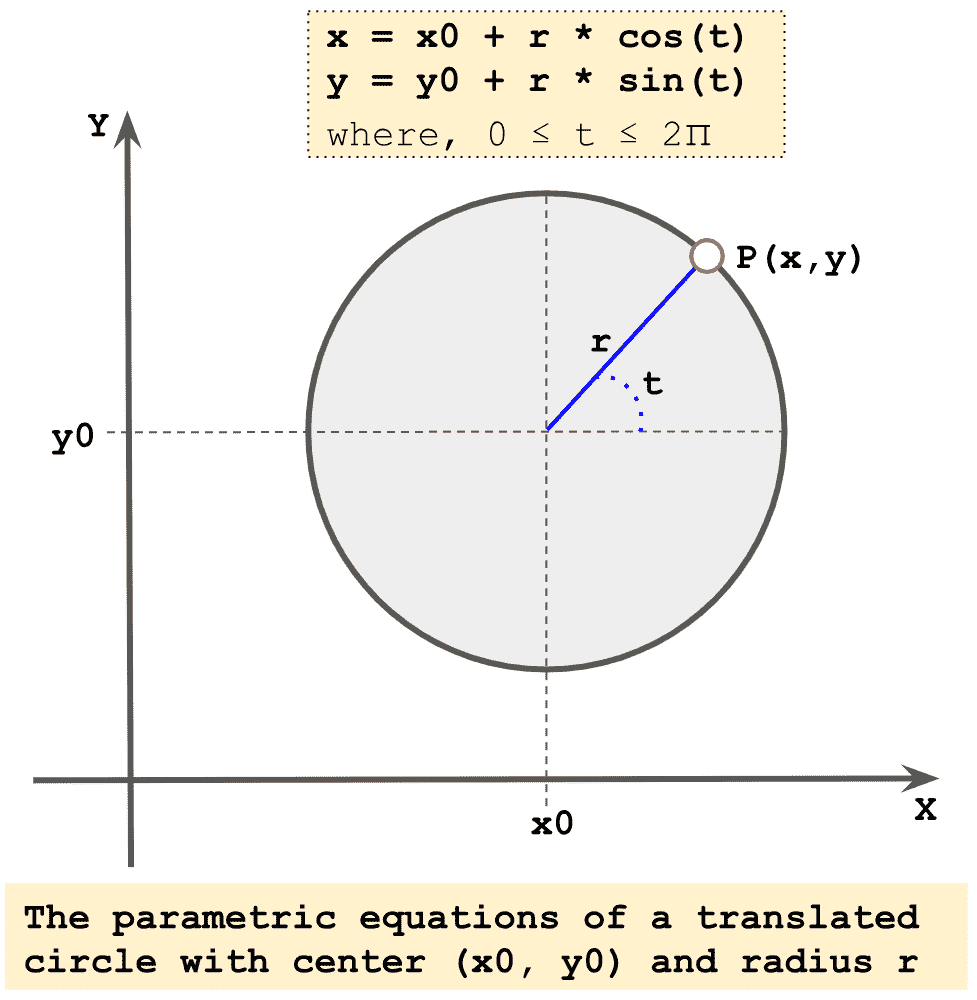radius = 300
center = (320, 320)

for x in (0, 30, 60, 90, 120, 150, 180, 210, 240, 270, 300, 330):
x_coordinate = center + radius * math.cos(x * 3.14/180)
y_coordinate = center + radius * math.sin(x * 3.14/180)
print("x: {} y: {}".format(round(x_coordinate), round(y_coordinate)))

for x in (0, 30, 60, 90, 120, 150, 180, 210, 240, 270, 300, 330):
x_coordinate = center + (radius - 20) * math.cos(x * 3.14/180)
y_coordinate = center + (radius - 20) * math.sin(x * 3.14/180)
print("x: {} y: {}".format(round(x_coordinate), round(y_coordinate)))


# 问题

1. 您应该正确配置哪个参数以绘制填充形状（例如，圆形或矩形）？
2. 您应该正确配置哪个参数以绘制抗锯齿线型？
3. 创建一条从(0, 0)开始到(512, 512)结束的对角线。
4. 使用所需的参数呈现文本Hello OpenCV
5. 使用 12 个点绘制一个多边形（圆形）。
6. 双击时使用鼠标事件和 Matplotlib 事件绘制一个矩形。
7. 尝试使用lenna.png图像作为背景绘制这个非常简单的模因生成器：